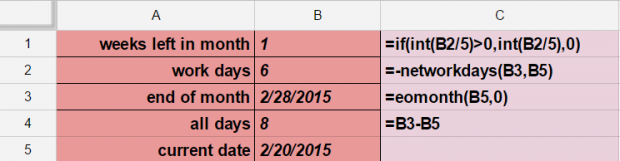# Google Sheets: How to Work with Dates

Posted February 6, 2015 by Walker Rowe in Google SheetsYou can use some basic date functions in Google Sheets to work with dates and use date math. Dates in spreadsheets are numbers, which means you can add and subtract them.  Do not think of them as strings of text such as “10 January 2015.” If you treat dates as strings of text, then using date math would be very complicated as you would have to isolate different parts of the date, convert that to a number, add or subtract from the number, and then convert it back to text. It is much easier to work just with dates as numbers and then use the formatting feature in Google Sheets to display the dates as you want them.

### Use Date Math with Google Sheets

This tutorial demonstrates how to use date math with the following computations:

• Count the number of work days between two days
• Count the total number of days between two dates
• Find the last day in the month
• Determine how many weeks are left in the month

Here is how to make each of these calculations and the formulas needed to do so.  Below the graphic, I explain the formulas.### Find the Number of Weeks Left in the Month

The function below uses the number of weekdays left in the month divided by five, since there are five days in the work week, to determine how many weeks are left in the month.  You could also take the number of days left in the month and divide by seven.  Note that it is necessary to use the int function to chop off the decimal point since, for example, it would not make much sense to say that there are 3.2 weeks left per month.

if(int(B2/5)>0,int(B2/5),0)

### Find the Number of Working Days between Two Dates

The networkdays functions find the number of workdays between two dates.  In order to exclude holidays, you would have to list them as the last argument in the forumla.

networkdays(B3,B5)

### Find the Last Day of the Month

The eomonth function shows the last day in the month.

eomonth(b5,0)

### Find the Number of Days between Two Dates

Since dates are numbers, you can just subtract one date from another.

B3-B5